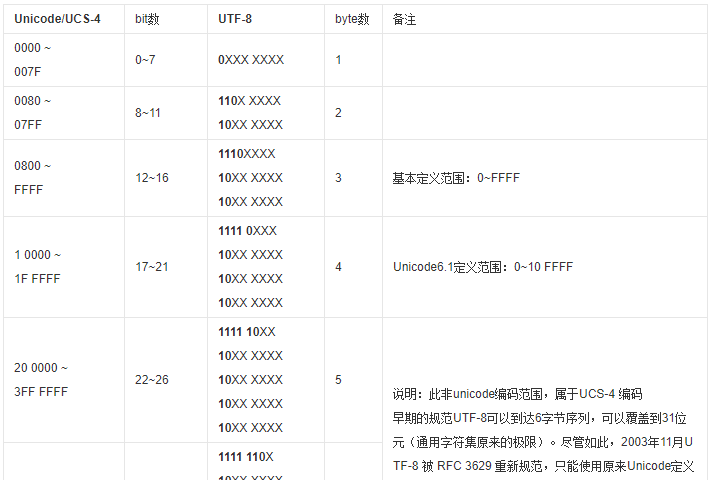# [个人题题解] 逆序输出

## 题目信息

【团队题目】 T50366 逆序输出

## 思路与解法First length
0xxx xxxx 1
10xx xxxx -1
110x xxxx 2
1110 xxxx 3
1111 0xxx 4
1111 1xxx -1

if(c<0) c+=256;


First length condition
0xxx xxxx 1 c<=128
110x xxxx 2 192<=c<=223
1110 xxxx 3 224<=c<=239
1111 0xxx 4 240<=c<=247
others -1 -

int classifyChar(int idx) {
int c=s[idx];
if(c<0) c+=256;
if(0<=c && c<=128) return idx+1;
if(192<=c && c<=223) return idx+2;
if(224<=c && c<=239) return idx+3;
if(240<=c && c<=247) return idx+4;
cerr<<"Wrong char: "<<c<<endl;
bool char_not_recognized=false;
assert(char_not_recognized);
return -1;
}


## 标准代码

#include<bits/stdc++.h>
using namespace std;

string s;
string t="";

int classifyChar(int idx) {
int c=s[idx];
if(c<0) c+=256;
if(0<=c && c<=128) return idx+1;
if(192<=c && c<=223) return idx+2;
if(224<=c && c<=239) return idx+3;
if(240<=c && c<=247) return idx+4;
cerr<<"Wrong char: "<<c<<endl;
bool char_not_recognized=false;
assert(char_not_recognized);
return -1;
}

int main() {
//freopen("input.txt","r",stdin);
//freopen("output.txt","w",stdout);
ios::sync_with_stdio(false);

getline(cin,s);
for(unsigned l=0;l<s.length();) {
int r=classifyChar(l);
t=s.substr(l,r-l)+t;
l=r;
}
cout<<t<<endl;
}



1.肖云帆说道：Google ChromeWindows 7

%%%